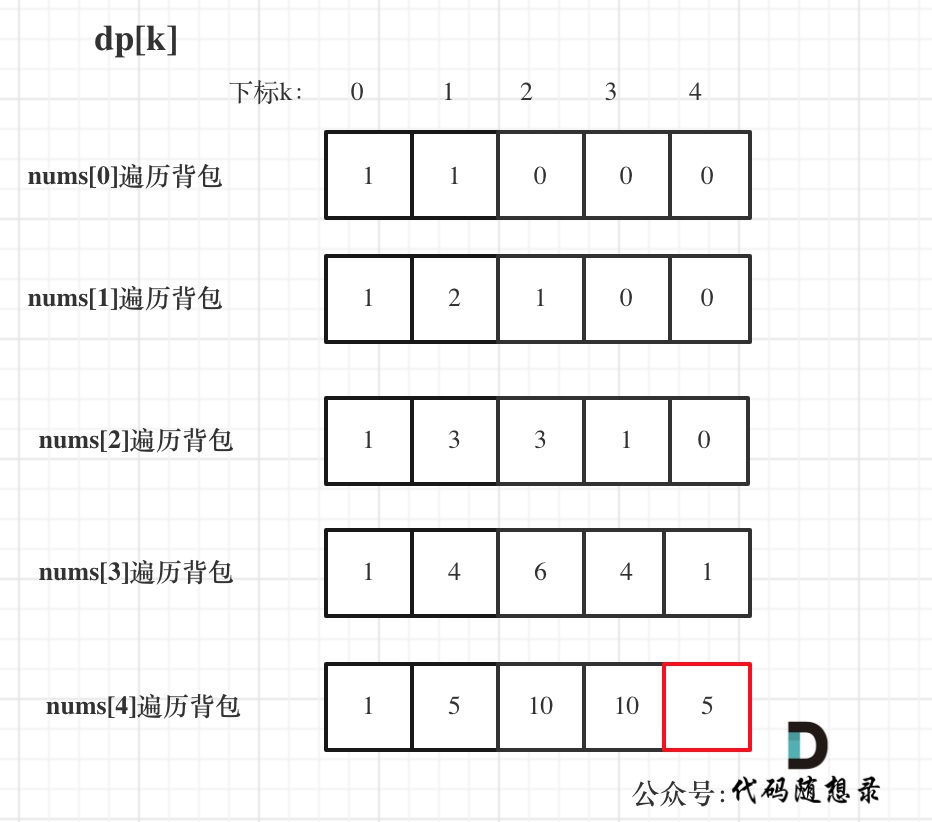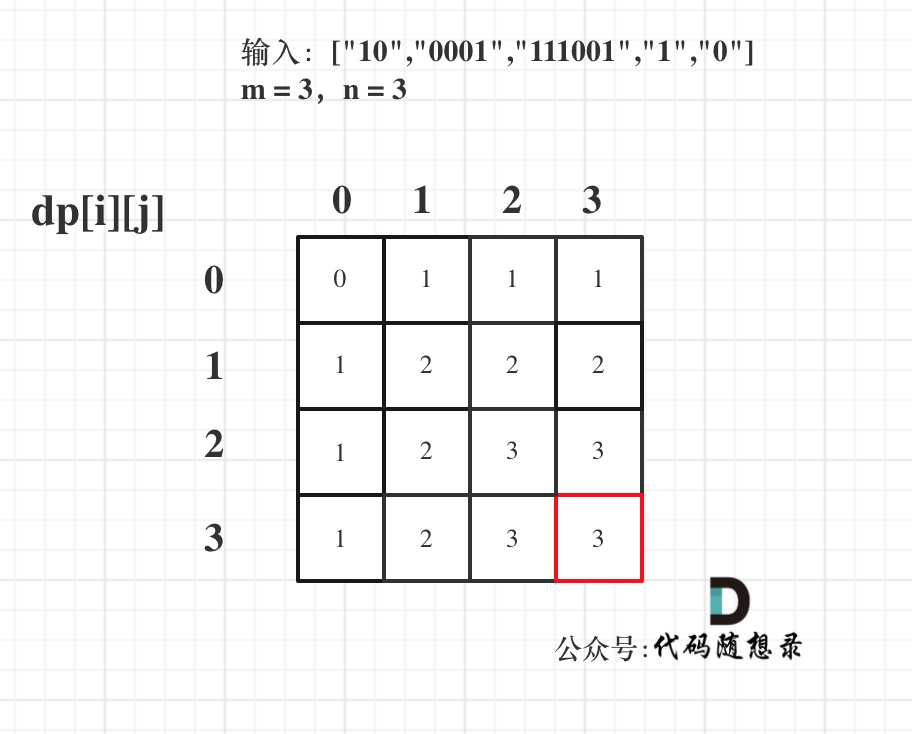# # 本周小结！（动态规划系列四）

## # 周一

S 和 sum都是固定的，那此时问题就转化为01背包问题（数列中的数只能使用一次）: 给你一些物品（数字），装满背包（就是x）有几种方法。

1. 确定dp数组以及下标的含义

dp[j] 表示：填满j（包括j）这么大容积的包，有dp[i]种方法

1. 确定递推公式

dp[i] += dp[j - nums[j]]

1. dp数组如何初始化

dp 初始化为1 ，dp[j]其他下标对应的数值应该初始化为0。

1. 确定遍历顺序

01背包问题一维dp的遍历，nums放在外循环，target在内循环，且内循环倒序。

1. 举例推导dp数组

bagSize = (S + sum) / 2 = (3 + 5) / 2 = 4

dp数组状态变化如下：## # 周二

1. 确定dp数组（dp table）以及下标的含义

dp[i][j]：最多有i个0和j个1的strs的最大子集的大小为dp[i][j]。

1. 确定递推公式

dp[i][j] = max(dp[i][j], dp[i - zeroNum][j - oneNum] + 1);

1. dp数组如何初始化

1. 确定遍历顺序

01背包一定是外层for循环遍历物品，内层for循环遍历背包容量且从后向前遍历！

1. 举例推导dp数组## # 周三

``````// 先遍历物品，再遍历背包
for(int i = 0; i < weight.size(); i++) { // 遍历物品
for(int j = weight[i]; j < bagWeight ; j++) { // 遍历背包容量
dp[j] = max(dp[j], dp[j - weight[i]] + value[i]);

}
}
``````
1
2
3
4
5
6
7

## # 周四

• 如果求组合数就是外层for循环遍历物品，内层for遍历背包。
• 如果求排列数就是外层for遍历背包，内层for循环遍历物品。

## # 总结